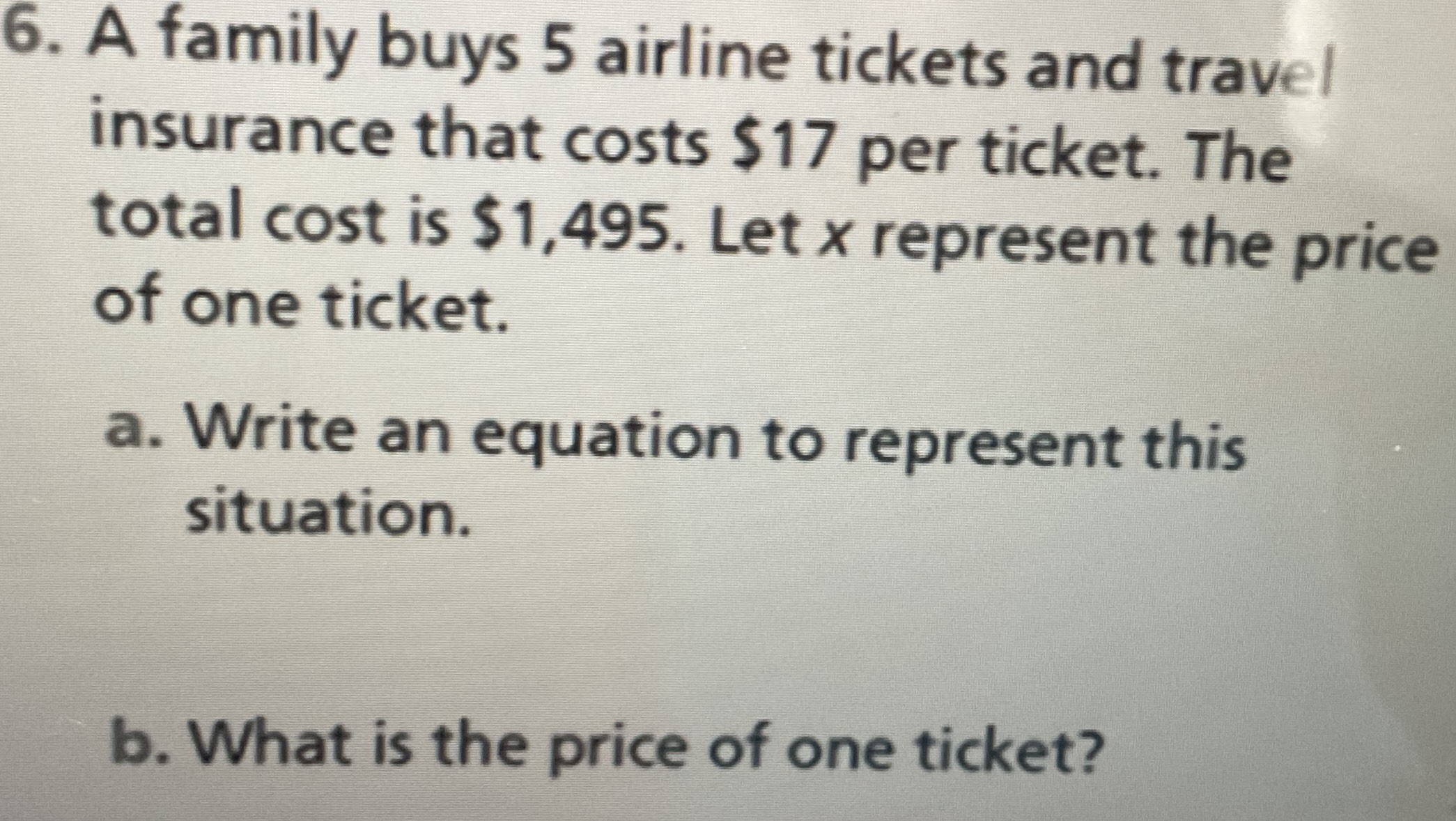### ¿Todavía tienes preguntas de matemáticas?

Pregunte a nuestros tutores expertos
Algebra
Pregunta6. A family buys $$5$$ airline tickets and travel insurance that costs $$\ 17$$ per ticket. The total cost is $$\ 1,495$$ . Let $$x$$ represent the price of one ticket. a. Write an equation to represent this situation. b. What is the price of one ticket?